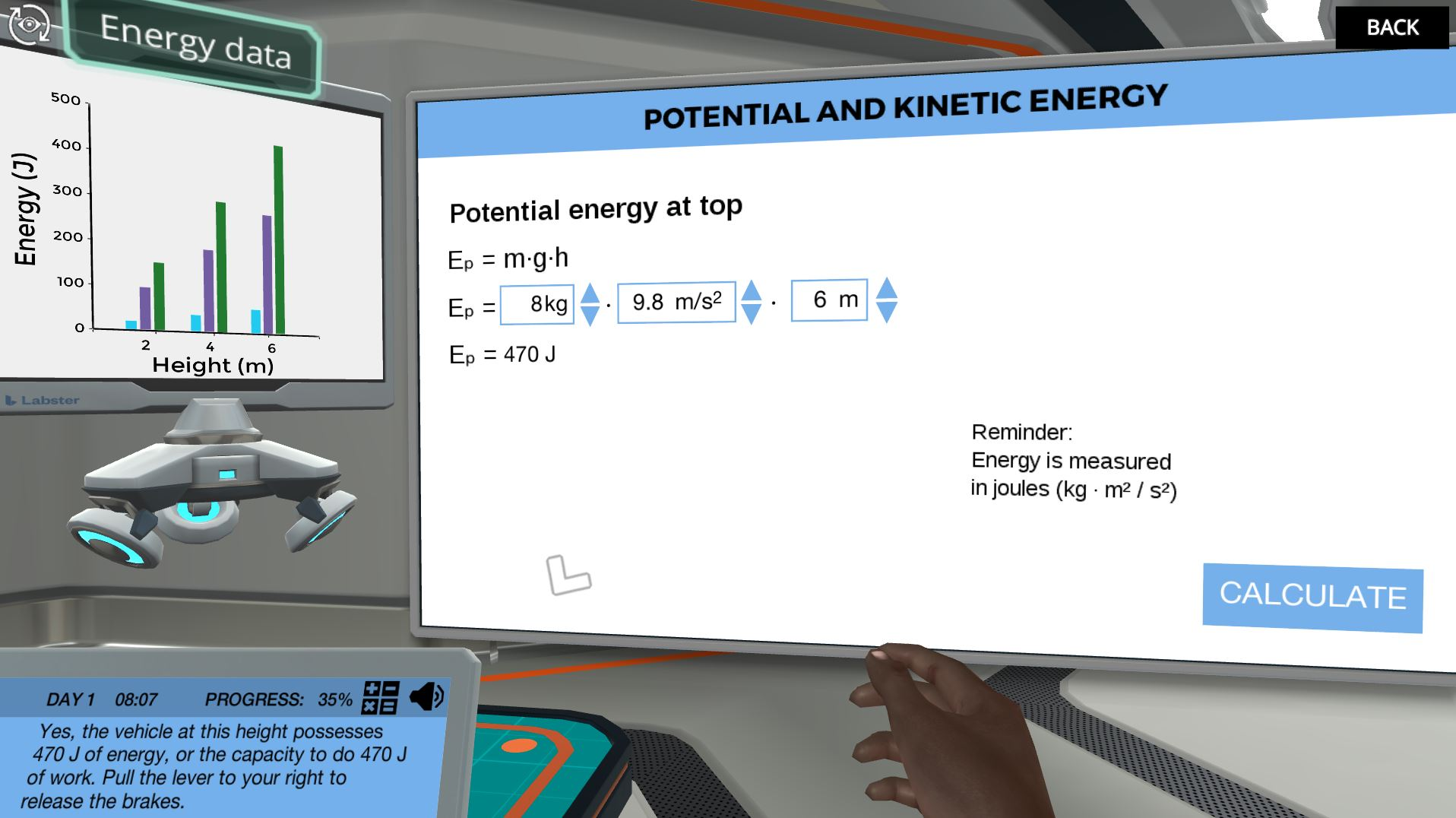Conservation of Energy: maximize the mechanical energy of a rollercoaster

Apply the concept of conservation of energy to make the Labster roller coaster ride faster and more exciting. Calculate potential, kinetic and mechanical energy using the formula board to determine how to best improve the roller coaster track.Try our lab safety simulation

Discover one of our 200+ learning simulations today

• Description
• Features

What does it take to make a roller coaster go 100 km/h? In this simulation, you will learn how manipulating the mass, height, and velocity of a roller coaster affect its overall potential energy and kinetic energy. You will apply the concept of conservation of energy to calculate the mechanical energy of the roller coaster at different positions.

Design a new roller coaster

First, familiarize yourself with the components of the equations for potential and kinetic energy. Next, experiment using different masses of vehicles at different starting positions to discover the effect of mass and height on potential energy, velocity and kinetic energy. Finally, understand how energy transformation can be applied to the calculation of mechanical energy and use this information to design the fastest, most exciting roller coaster.

Calculate potential, kinetic and mechanical energy

Students will become comfortable with the components of the formulae for potential and kinetic energy and how these components are related to joules. By measuring energy in joules and converting from one type to another, students will discover how energy remains constant in an isolated system. Students will have the freedom to experiment with different masses of vehicles at each height to determine which combination fits their initial potential energy desire. Pulling the lever releases the rollercoaster and tracks the velocity, which can then be used to calculate the kinetic and mechanical energy.

Make the ride go 100 km/h

Choose the best vehicle and starting position then change the track by adding loops, dips and even an electric motor. Can you reach 100 km/h?

Get started now

Apply the concept of conservation of energy to make the Labster roller coaster ride faster and more exciting. Calculate potential, kinetic and mechanical energy using the formula board to determine how to best improve the roller coaster track.

Techniques in lab
Manipulate formulae for potential, kinetic and mechanical energy
Learning objectives

At the end of this simulation, you will be able to...

• Define the mechanical energy of an isolated system
• Separate conservative from nonconservative forces
• Define the mechanical energy of a body
• Explain the conservation of mechanical energy
• Apply conservation of energy to describe the movement of a body
• Use the equations for kinetic and potential energy, related to velocity and displacement
• Identify sources of energy and energy transformation
Simulation features

Length: 40
Accessibility mode: Not available
Languages: English (United States)

NGSS

HS-PS3-1, HS-PS3-3, HS-PS3-4, HS-ETS1-2

IB

2.3 Work, energy and power

AP

4.1 Open and Closed Systems: Energy

4.2 Work and Mechanical Energy

4.3 Conservation of Energy, the Work-Energy Principle, and Power